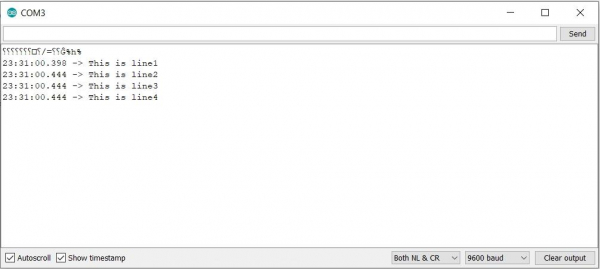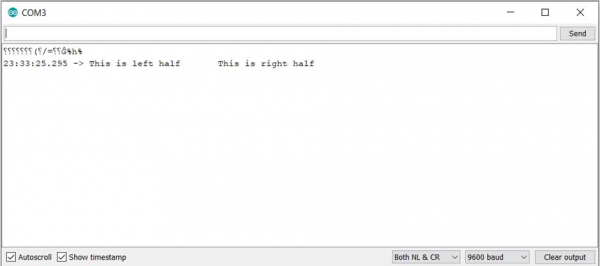# Print new line and tab in Arduino

In order to print a newline, you can either introduce the '

An example code is given below −

## Example

void setup() {
// put your setup code here, to run once:
Serial.begin(9600);
Serial.println();
Serial.print("This is line1This is line2");
Serial.println("This is line3");
Serial.println("This is line4");
}
void loop() {
// put your main code here, to run repeatedly:

}

The Serial Monitor output for the above code is −

## OutputIn order to add a tab space, you can introduce '\t' in your code.

An example code is given below −

## Example

void setup() {
// put your setup code here, to run once:
Serial.begin(9600);
Serial.println();
Serial.print("This is left half\tThis is right half");
}
void loop() {
// put your main code here, to run repeatedly:

}

The corresponding Serial Monitor output is −

## Output